July 17, 2019

# Growth of Functions

• Order of growth of the running time of an algorithm
• Characterizes an algorithm’s efficiency
• Allows comparing relative performance of algorithms
• With large enough input sizes, in terms of $$n$$
• Merge sort $$\theta(n \lg n)$$ beats insertion sort $$\theta(n^2)$$

# Growth of Functions

• We study large enough input sizes to make relevant the order of growth of the running time
• We study the asymptotic efficiency of algorithms
• How running time of an algorithm increases with the size of the input in the limit, as input size increases without bound
• An asymptotically more efficient algorithm is usually the best choice for all but very small inputs

# Asymptotic Notation

• Notation to describe asymptotic running time of an algorithm
• Defined as functions with domain as $$N = \{0, 1, 2, \dots\}$$
• Describe worst case running time of $$T(n)$$
• Usually defined only for integer input sizes
• Careful not to abuse asymptotic notation

# Asymptotic Notation, Functions and Running Times

• Remember we characterize running time of algorithms with functions
• Insertion sort: $$\Theta(n^2)$$
• After abstraction from $$an^2 + bn + c$$
• $$\Theta(n^2)$$ stands for function $$an^2 + bn + c$$ as the worst case running time of insertion sort
• Asymptotic notation can also characterize other aspects of algorithms
• i.e. amount of space

# $$\Theta$$-notation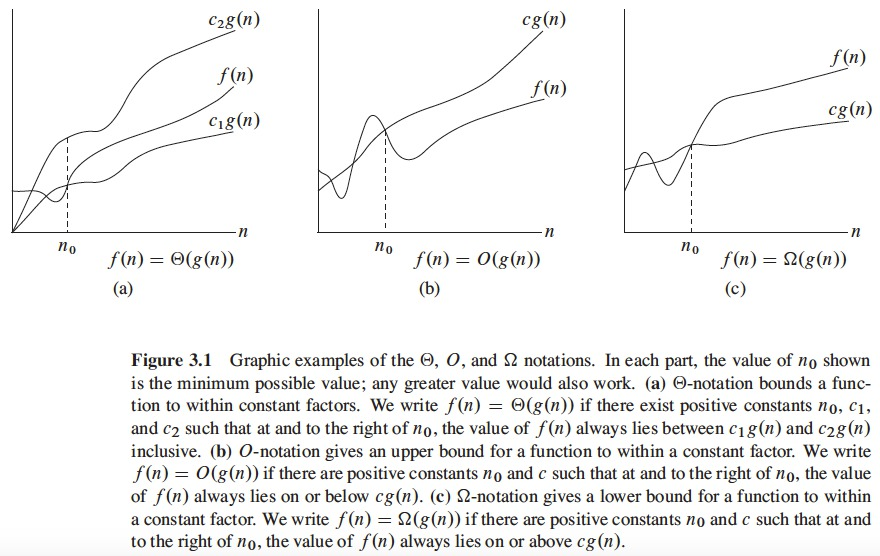# $$\Theta$$-notation

• What does $$T(n) = \Theta(n^2)$$ means as worst-case running time for insertion sort?
• For a given function $$g(n)$$, we denote by $$\Theta(g(n))$$ the set of functions

$$\Theta(g(n)) = \{f(n):$$ there exist positive constants $$c_1$$, $$c_2$$, and $$n_0$$ : $$0 \leq c_1g(n) \leq f(n) \leq c_2g(n)$$ $$\forall$$ $$n \geq n_0\}$$.

# $$\Theta$$-notation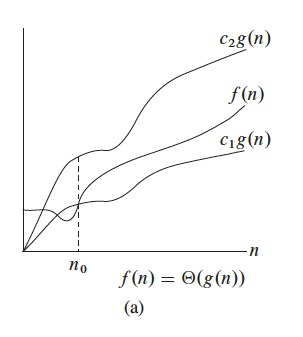# $$\Theta$$-notation

• A function $$f(n)$$ belongs to the set $$\Theta(g(n))$$ if there exist positive constants $$c_1$$ and $$c_2$$ such that it can be “sandwiched” between $$c_1g(n)$$ and $$c_2g(n)$$, for sufficiently large $$n$$.
• $$\Theta(g(n))$$ is a set, then, $$f(n) \in \Theta(g(n))$$, but we write: $$f(n) = \Theta(g(n))$$
• For all $$n \geq n_0$$, $$f(n)$$ is equal to $$g(n)$$ to within a constant factor
• $$g(n)$$ is an asymptotically tight bound for $$f(n)$$
• By definition, $$\Theta(g(n))$$ requires every member $$f(n) \in \Theta(g(n))$$ be asymptotically nonnegative
• $$f(n)$$ nonnegative when $$n$$ is sufficiently large

# $$\Theta$$-notation

• Using the formal definition
• Example of using the $$\Theta$$-notation
• In function $$\frac{1}{2}n^2 - 3n = \Theta(n^2)$$
• Determine positive constants $$c_1$$, $$c_2$$, and $$n_0$$ such that $$c_1n^2 \leq \frac{1}{2}n^2 - 3n \leq c_2n^2$$
• dividing by $$n^2$$, $$c_1 \leq \frac{1}{2} - \frac{3}{n} \leq c_2$$
• For case $$c_1 \leq 1/2 - 3/n$$
• Because $$c_1$$ is a positive constant, then, $$0 < 1/2 - 3/n$$, then $$n > 6$$
• then, if $$n_0 = 7$$ then $$c_1 \leq 1/2 - 3/7$$, which is equal to $$c_1 \leq 1/14$$. Let $$c_1 = 1/14$$

# $$\Theta$$-notation

• Determine positive constants $$c_1$$, $$c_2$$, and $$n_0$$ such that $$c_1n^2 \leq \frac{1}{2}n^2 - 3n \leq c_2n^2$$
• For case $$1/2 - 3/n \leq c_2$$, when $$n \rightarrow \infty$$ then, $$1/2 - 3/n \rightarrow 1/2$$, then, $$c_2 = 1/2$$
• For $$c_1 = 1/14$$, $$c_2 = 1/2$$ and $$n_0 = 7$$, it holds that $$f(n) \in \Theta(n^2)$$ or $$\frac{1}{2}n^2 - 3n = \Theta(n^2)$$
• other constants exist!

# $$O$$-notation

• The $$O$$ notation denotes an asymptotic upper bound
• For a function $$g(n)$$ we denote $$O(g(n))$$ and say: big-oh of g of n, or oh of g of n
• $$O(g(n))$$ also refers to a set of functions
• $$O(g(n)) = \{ f(n) :$$ there exist positive constants $$c$$ and $$n_0$$ such that $$0 \leq f(n) \leq cg(n)$$ $$\forall$$ $$n \geq n_0 \}$$.
• Use $$O$$-notation to give upper bound on a function, to within a constant factor
• Note that $$f(n) = \Theta(g(n))$$ implies $$f(n) = O(g(n))$$
• $$\Theta$$-notation is stronger than $$O$$-notation

# $$O$$-notation

• Given a function $$g(n)$$, we denote as $$O(g(n))$$ to the set of functions such that: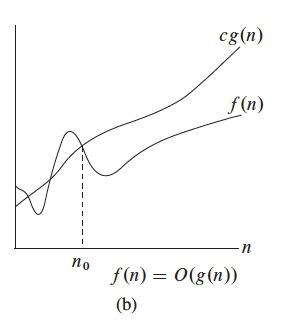# $$O$$-notation

• We often describe the running time of an algorithm by inspecting the algorithm’s overall structure
• We use $$O$$-notation
• A doubly nested loop structure shows an $$O(n^2)$$ upper bound on the worst case running time

# $$\Omega$$-notation

• $$\Omega$$-notation provides an asymptotic lower bound
• For a function $$g(n)$$, we denote $$\Omega(g(n))$$ and say: big-omega of g of n or omega of g of n
• $$\Omega(g(n))$$ also refers to a set of functions
• $$\Omega(g(n)) = \{ f(n) :$$ there exist positive constants $$c$$ and $$n_0$$ such that $$0 \leq cg(n) \leq f(n)$$ $$\forall$$ $$n \geq n_0 \}$$. Use $$\Omega$$-notation to give lower bound on a function, to within a constant factor
• Note that $$f(n) = \Theta(g(n))$$ also implies $$f(n) = \Omega(g(n))$$
• $$\Theta$$-notation is stronger than $$\Omega$$-notation

# $$\Omega$$-notation

• Given a function $$g(n)$$, we denote as $$\Omega(g(n))$$ to the set of functions such that: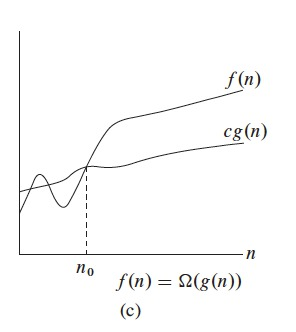# $$\Omega$$-notation

• Theorem 3.1
• For any two functions $$f(n)$$ and $$g(n)$$, we have $$f(n) = \Theta(g(n))$$ if and only if $$f(n) = O(g(n))$$ and $$f(n) = \Omega(g(n))$$.

# $$\Omega$$-notation

• If we say the running time of an algorithm is $$\Omega(g(n))$$, we mean:
• No matter what particular input of size n is chosen for each value of n, the running time on that input is at least a constant times $$g(n)$$ for sufficiently large $$n$$
• We can also mean best-case times, as in insertion sort is $$\Omega(n)$$.
• Insertion Sort belogs to both, $$\Omega(n)$$ and $$O(n^2)$$

# Asymptotic Notation in Equations and Inequalities

• In $$n = O(n^2)$$, the $$=$$ sign means set membership: $$n \in O(n^2)$$
• When we use asymptotic notation in a formula, we interpret it as representing a function for which we don’t care about its name, only its order
• In $$2n^2 + 3n + 1 = 2n^2 + \Theta(n)$$ means $$2n^2 + 3n + 1 = 2n^2 + f(n)$$, where $$f(n)$$ is a function in the set $$\Theta(n)$$
• Example:
• $$2n^2 + 3n + 1 = 2n^2 + \Theta(n) = \Theta(n^2)$$.

# $$o$$-notation

• The upper bound $$O-notation$$ may or may not be asymptotically tight
• $$2n^2 = O(n^2)$$ is asymptotically tight
• $$2n = O(n^2)$$ is not asymptotically tight
• We use $$o-notation$$ to denote an upper bound that is not asymptotically tight
• We define $$o(g(n))$$ and say little-oh of g of n as the set $$o(g(n)) = \{ f(n) :$$ for any positive constant $$c > 0$$, there exists a constant $$n_0 > 0$$ such that $$0 \leq f(n) < cg(n)$$ $$\forall$$ $$n \geq n_0 \}$$.
• Example, $$2n = o(n^2)$$ but $$2n^2 \neq o(n^2)$$.

# $$\omega$$-notation

• $$\omega$$-notation is to $$\Omega$$-notation as $$o$$-notation is to $$O$$-notation
• Use $$\omega$$-notation to denote a lower bound that is not asymptotically tight
• $$f(n) \in \omega(g(n))$$ if and only if $$g(n) \in o(f(n))$$.
• Formally
• $$\omega(g(n)) = \{ f(n) :$$ for any positive constant $$c > 0$$, there exists a constant $$n_0 > 0$$ such that $$0 \leq cg(n) < f(n)$$ $$\forall$$ $$n \geq n_0 \}$$.
• $$n^2/2 = \omega(n)$$
• $$n^2/2 \neq \omega(n^2)$$

# Comparing functions

• Many relational properties of real numbers also apply to asymptotic comparisons

# Comparing functions

• Properties of Asymptotic Functions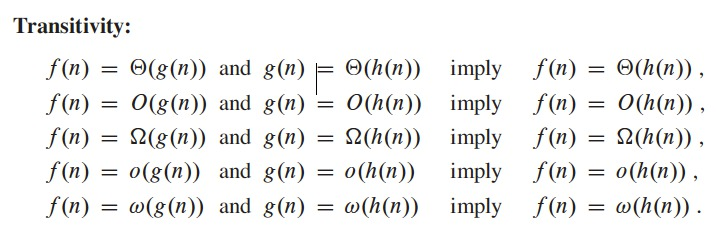# Comparing functions

• Properties of Asymptotic Functions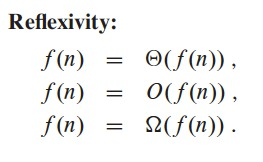# Comparing functions

• Properties of Asymptotic Functions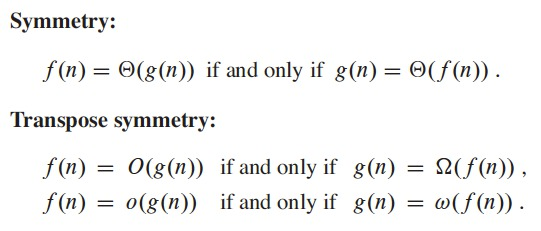# Comparing functions

• Analogy between asymptotic comparison of 2 functions $$f$$ and $$g$$ and comparison of 2 real numbers $$a$$ and $$b$$: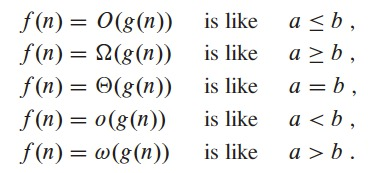# Comparing functions

• Trichotomy: for any 2 real numbers $$a$$ and $$b$$, exactly one of the following must hold: $$a < b$$, $$a = b$$, or $$a > b$$
• NOT all functions are asymptotically comparable
• Given 2 functions $$f(n)$$ and $$g(n)$$, it could be that neither $$f(n) = O(g(n))$$ nor $$f(n) = \Omega(g(n))$$ holds

# Assignment

• Review section 3.2, Standard notations and common functions
• Review the loop invariant proof for the MERGE procedure used in MergeSort (by the end of section 2.3.1 of the textbook)
• Problem 2-2 Correctness of bubblesort
• Problem 3-2 Relative asymptotic growths